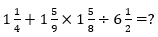Dear Students,

If talked about banking exams, Quant Section is considered to be one of the most difficult sections and so, gives heebie-jeebies to many. The questions asked in this section are calculative and very time-consuming and if you do not practice it well, it can make your blood run cold during the exam. The IBPS Clerk Mains Exam is scheduled to be held on the 20th of January 2019. Adda247 is here with "Practice Marathon for Clerk Mains 2018"  with practice questions on all the topics that are likely to be asked in the exam. Today is Day 5 of IBPS Clerk Mains Practice Marathon, attempt the quiz and win this marathon against lakhs of IBPS Clerk Candidates.

Watch The Video Solutions

Q1. A policeman starts to chase a thief. When the thief goes 10 steps the policeman moves 8 steps and 5 steps of the policeman are equal to 7 steps of the thief. The ratio of the speeds of the policeman and the thief is:
25 : 28
25 : 26
28 : 25
56 : 25
14:27

Q2. Stanic and Paul take a piece of work for Rs. 28,800. One alone could do it in 36 days, the other in 48 days. With the assistance of an expert, they finish it in 12 days. How much remuneration the expert should get?
Rs. 10000
Rs. 18000
Rs. 16000
Rs. 12000
Rs. 15000

Q3. A and B together can complete a job in 8 days. Both B and C, working alone can finish the same job in 12 days, A and B commence work on the job, and work for 4 days, where upon A leaves, B continues for 2 more days, and then he leaves too, C now starts working, and finishes the job. How many days will C require?
5 days
8 days
3 days
4 days
9 days

Q4. In how many different ways can 4 boys and 3 girls be arranged in a row such that all boys stand together and all the girls stand together?
75
576
288
24
121

Q5. In how many different ways can the letters of the word ‘HAPPY’ be arranged?
120
140
60
70
45

Directions (6-10): Read the given data carefully and answer the given question

Pie chart shows the percentage distribution of time taken by 8 different persons namely A, B,C, D,E,F,G and H to reach a particular point but the starting point is not same and total time taken by all of them together is 500 hr. (partly by car and remaining by train)
The line graph shows percentage distribution distance travelled by car out of total distance travelled by them individually.Q6. Find speed of E by train, if the speed of car is 60 km/hr and time taken by car and train are in the ratio of 4 : 3.
50 km/hr
45 km/hr
53 ⅓km/hr
56 ⅔ km/hr
51 ⅔ km/hr

Q7. Find the speed of D by car. If the speed by train is 22 km/hr and time taken by car is 50% less than the train.
45 km/hr
54 km/hr
36 km/hr
65 km/hr
48 km/hr

Q8. If the distance travelled by B, C and D together and E,F,G and H together is same. Then find the ratio of average speed of B,C and D together and E,F,G and H together.
6 : 5
8 : 7
7 : 8
5 : 6
7 : 9

Q9. Average speed of A and C are in the ratio of 4 : 3 and C have travelled 500 km more than A. Find the speed of A by car if the speed of A by train is 120 km/hr.
80 km/hr
60 km/hr
65 km/hr
75 km/hr
None of these

Q10. Total distance travelled by G is 2250 and the speed by train is 45 km/hr. Find the speed of G by car.
50 km/hr
60 km/hr
70 km/hr
40 km/hr
80 km/hr

Directions (11-15): What should come in place of question mark (?) in the following questions?

Q11. √1225÷ ∛343× 45% of 760 = ?
1170
1760
1510
1710
1050

Q12. [(5√7+√7)×(4√7+8√7)]-(19)²=?
143
72√7
134
70√7
143√7

Q13.Q14. (21% of 1326) – (17% of 932) = ?
120.02
206.05
240.04
120.20
204.25

Q15. (?)³÷32=54
18
12
14
16
11

You May also like to Read: